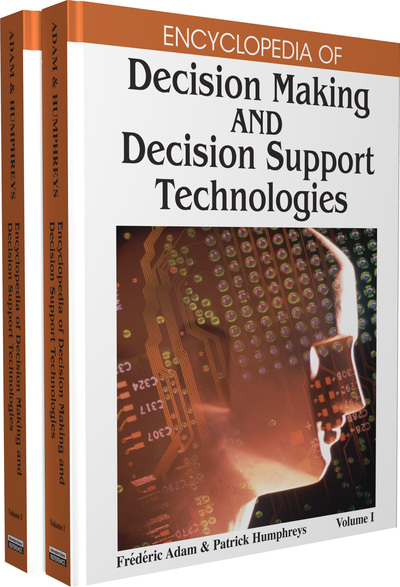# Uncertainty and Vagueness Concepts in Decision Making

Georg Peters (Munich University of Applied Sciences, Germany)
DOI: 10.4018/978-1-59904-843-7.ch101

## Abstract

One of the main challenges in decision making is how to deal with uncertainty and vagueness. The classic uncertainty concept is probability, which goes back to the 17th century. Possible candidates for the title of father of probability are Bernoulli, Laplace, and Pascal. Some 40 years ago, Zadeh (1965) introduced the concept of fuzziness, which is sometimes interpreted as one form of probability. However, we will show that the terms fuzzy and probability are complementary. Recently, in the beginning of the ’80s, Pawlak (1982) suggested rough sets to manage uncertainties. The objective of this article is to give a basic introduction into probability, fuzzy set, and rough set theory and show their potential in dealing with uncertainty and vagueness. The article is structured as follows. In the next three sections we will discuss the basic principles of probability, fuzzy sets, and rough sets, and their relationship with each other. The article concludes with a short summary.

## Key Terms in this Chapter

Granular Computing: The idea of granular computing goes back to Zadeh (1979). The basic idea of granular computing is that an object is described by a bunch of values in possible dimensions like indistinguishability, similarity, and proximity. If a granular is labeled by a linguistic expression it is called a linguistic variable.

Hybridization: This is a combination of methods like probabilistic, fuzzy, rough concepts, or neural nets, for example, fuzzy-rough, rough-fuzzy or probabilistic-rough, or fuzzy-neural approaches.

Soft Computing: In contrast to hard computing, soft computing is a collection of methods (fuzzy sets, rough sets, neutral nets, etc.) for dealing with ambiguous situations like imprecision and uncertainty, for example, human expressions like “high profit at reasonable risks”. The objective of applying soft computing is to obtain robust solutions at reasonable costs.

Rough Set Theory: Rough set theory was introduced by Pawlak in 1982. The central idea of rough sets is that some objects are distinguishable while others are indiscernible from each other.

Linguistic Variable: A linguistic variable is a linguistic expression (one or more words) labeling an information granular. For example, a membership function is labeled by expressions like “hot temperature” or “rich customer”.

Rough Approximations: In rough set theory, a set is described by a lower and an upper approximation. The objects in the lower approximation surely belong to the set while the objects in the upper approximation may belong to the set.

Membership Function: A membership function shows the membership degrees of a variable to a certain set. For example, a temperature t=30° C belongs to the set hot temperature with a membership degree ?HT(30°)=0.8. The membership functions are not objective but context and subject dependent.

Fuzzy Set Theory: Fuzzy set theory was introduced by Zadeh in 1965. The central idea of fuzzy set theory is that an object belongs to more than one set simultaneously. The closeness of the object to a set is indicated by membership degrees.

## Complete Chapter List

Search this Book:
Reset